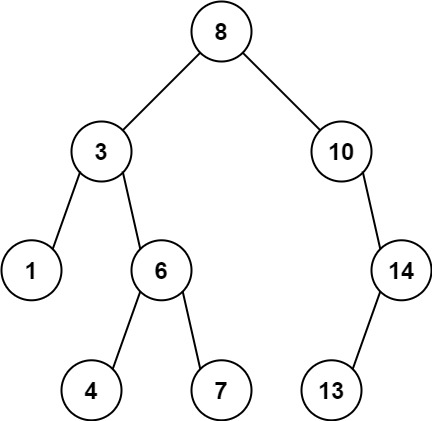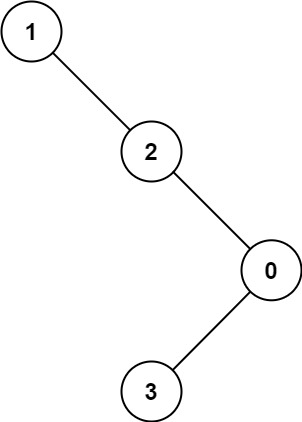# 1026. Maximum Difference Between Node and Ancestor

Medium
Given the `root` of a binary tree, find the maximum value `v` for which there exist different nodes `a` and `b` where `v = |a.val - b.val|` and `a` is an ancestor of `b`.
A node `a` is an ancestor of `b` if either: any child of `a` is equal to `b` or any child of `a` is an ancestor of `b`.
Example 1:Input: root = [8,3,10,1,6,null,14,null,null,4,7,13]
Output: 7
Explanation: We have various ancestor-node differences, some of which are given below :
|8 - 3| = 5
|3 - 7| = 4
|8 - 1| = 7
|10 - 13| = 3
Among all possible differences, the maximum value of 7 is obtained by |8 - 1| = 7.
Example 2:Input: root = [1,null,2,null,0,3]
Output: 3
Constraints:
• The number of nodes in the tree is in the range `[2, 5000]`.
• `0 <= Node.val <= 10^5`

### 解題

/**
* Definition for a binary tree node.
* type TreeNode struct {
* Val int
* Left *TreeNode
* Right *TreeNode
* }
*/
func maxAncestorDiff(root *TreeNode) int {
ans := 0
var helper func(*TreeNode, int, int)
helper = func(root *TreeNode, max int, min int) {
if root == nil {
return
}
if abs(max - root.Val) > ans {
ans = abs(max - root.Val)
}
if abs(min - root.Val) > ans {
ans = abs(min - root.Val)
}
nmax := max
nmin := min
if root.Val > nmax { nmax = root.Val }
if root.Val < nmin { nmin = root.Val }
helper(root.Right, nmax, nmin)
helper(root.Left, nmax, nmin)
}
helper(root, root.Val, root.Val)
return ans
}
func abs(a int) int {
if a < 0 {
return -a
}
return a
}